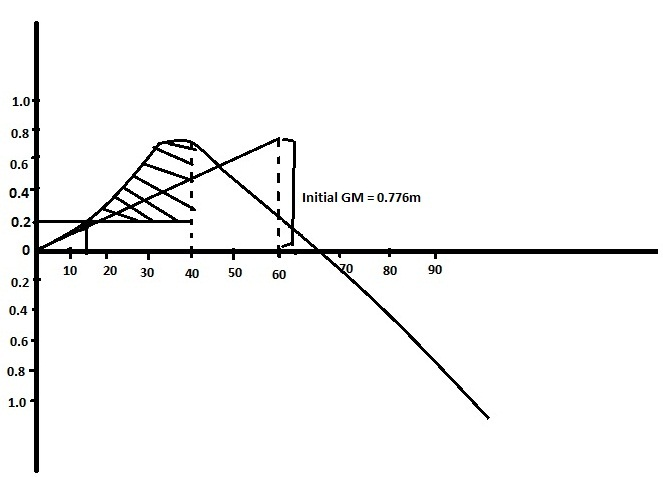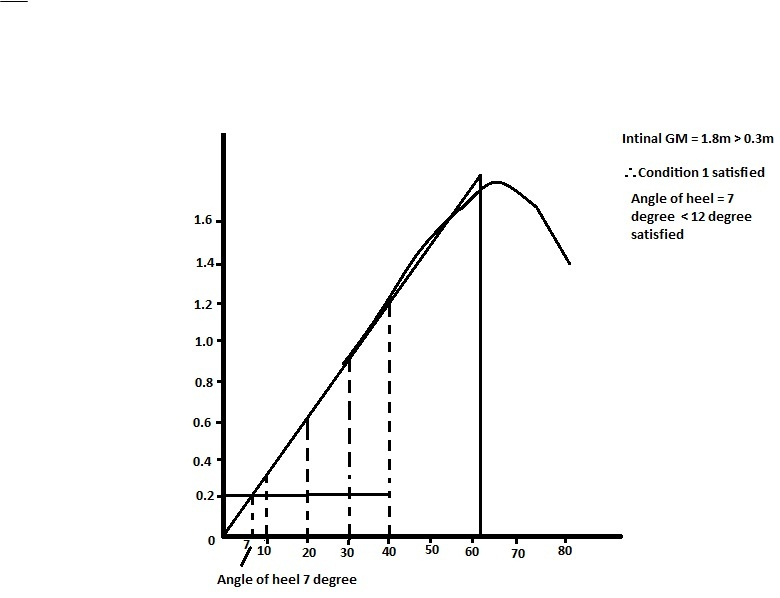Miscelleneous

# C L DUBEY – EXERCISE – 11 (Grain Stability)

###### Solution –

Given VHM = 3960m4
Weight heeling moment = 3960/1.2
= 3300m4
λ0 = 0.2m, λ40 = 0.16m

• GM = 0.776m

Therefore, condition 1 satisfied

• Angle of heel = 120 = Marginal.Heel Ordinate SM Product 0 0.0 1 0 10 0.15 4 0.60 20 0.33 2 0.66 30 0.645 4 2.28 40 0.610 1 0.610 4.45

Area = 10/3 ⨯ 4.45/57.3

Area of triangle = 0.5 ⨯ 12 ⨯ 0.19/57.3

Area of trapezium = 0.5 ⨯ (0.19 + 0.16) ⨯ 28/57.3

Residual area = 0.16m radian ˃ 0.075
Therefore, condition 3 satisfied.

###### Solution –

Given λ0 = 0.275, λ40 = 0.22Heel Ordinate SM Product 0 0 1 o 10 0.35 4 1.4 20 0.764 2 1.53 30 1.227 4 4.91 40 1.551 1 1.55 SOP = 9.30

Area of triangle = 0.5 ⨯ 7 ⨯ 0.265/57.3

Area of trapezium = 0.5 ⨯ (0.265 + 0.22) ⨯ 33/57.3

Therefore, condition 3 satisfied